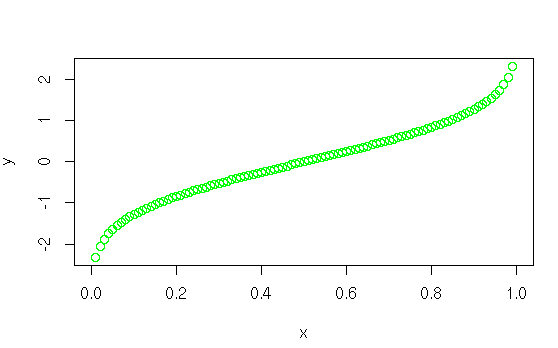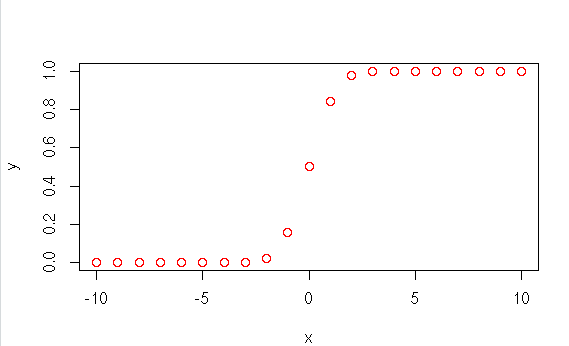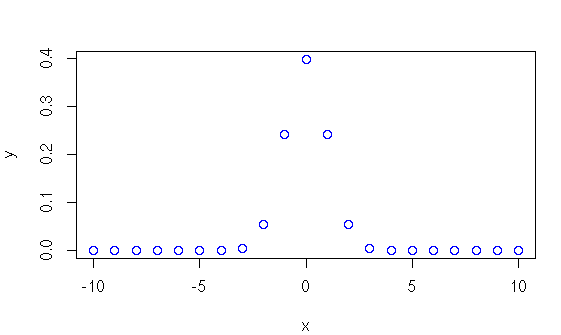• #5, First Floor, 4th Street Dr. Subbarayan Nagar Kodambakkam, Chennai-600 024 Landmark : Samiyar Madam
• pro@slogix.in
• +91- 81240 01111

### How to implement normal distribution in R?

###### Description

To implement the normal distribution using R programming.

#### Four main functions in normal distribution:

dnorm(x, mean= sd= )

Gives height of the probability distributionat each point for a given mean andstandard deviation.

pnorm(x, mean= sd= ) Gives the probabi lity of a normally distributed random number to be less that the value of a given number. qnorm(p, mean= sd= )

It takes the probability value and givesa number whose cumulative value matchesthe probability value. rnorm(n, mean= sd= )

Used to generate random numberswhose distribution is normal.

x -- vector

mean -- Mean value of the sample data.

It's default value is zero.

prob -- The standard deviation. It's default value is 1.

###### Sapmle Code

#Normal Distribution
#dnorm
x<-seq(-10,10,by = 1)
y<-dnorm(x,mean=0,sd=1)
plot(x,y,col=”blue”)

#pnorm
y<-pnorm(x,mean=0,sd=1)
plot(x,y,col=”red”)

#qnorm
x<-seq(0,1,by=0.01)
y<-qnorm(x,mean=0,sd=1)
plot(x,y,col=”green”)

#rnorm
x<-rnorm(100)
hist(x,col=285)

###### Screenshots